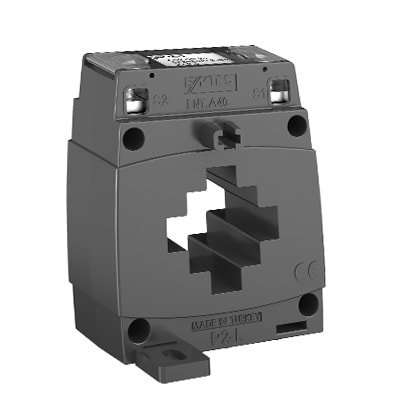# ENT.A40 Series

 Secondary Current 5A 1A Primary Current (A) Nominal Power(VA) Class Class 1 0,5 0,2 0,2S 1 0,5 0,2 0,2S 75 1 X X X X ✓ X X X 80 1 X X X X ✓ X X X 100 1,25 X X X X ✓ X X X 125 2,5 X X X X ✓ X X X 150 2,5 X X X X ✓ X X X 200 2,5 X X X X X ✓ X X 250 2,5 X X X X X ✓ X X 300 2,5 X X X X X ✓ X X 10 X ✓ X X X X X X 400 5 X X ✓ X X ✓ X X 10 X ✓ X X X X X X 500 5 X X ✓ X X ✓ X X 10 X ✓ X X X X X X 600 5 X X X ✓ X ✓ X X 10 X ✓ ✓ X X X X X 750 7,5 X X X ✓ X X X X 10 X X ✓ X X X X 800 10 X X ✓ ✓ X X X X
OTHER PRODUCTS
SUPPORT AND DOCUMENTS
Get Offer
Let Us Call You